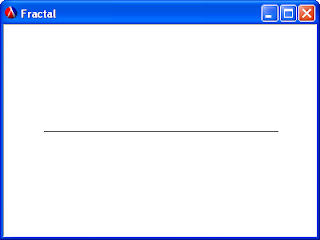## Wednesday, April 25, 2007

### A quick diversion

When I was in high school I created a little fractal similar to the Koch curve and programmed it in BASIC.

The basic algorithm replaces a line segmentwith nine line segments like so:You can repeat the process arbitrarily, and you get pretty curves looking like this:It was the first hard program I ever wrote (made especially hard by the fact that I didn't know about datatypes, recursion, or even basic procedural abstraction, really), and I was really proud of my 15-year-old self. Every once in a while I think of another cute way to program it, and it makes me nostalgic. Here's a nice little one.

A direction is one of four symbols, 'N, 'E, 'S, or 'W. An orientation is one of two symbols, 'CW or 'CCW.

To rotate a direction 90 degrees clockwise or counter-clockwise, keep a "clock" of the cardinal directions, and rotate the clock index:
`(define clock '(N E S W));; rotate : orientation * direction -> direction(define (rotate or dir)  (let ([shift (if (eq? or 'CW) add1 sub1)])    (list-ref clock (modulo (shift (list-index (lambda (x)                                                 (eq? x dir))                                               clock))                            4))))`
Then computing a fractal iteration is decomposed into two stages. The first stage computes the list of directions for each line segment in sequence. It recursively computes each iteration by replacing each direction in the previous iteration with a sequence of nine rotated directions.
`;; directions-for : nat * direction -> (listof direction)(define (directions-for n dir)  (if (zero? n)      (list dir)      (append-map (lambda (d)                    (list d                          (rotate 'CCW d)                          d                          (rotate 'CW d)                          d                          (rotate 'CW d)                          d                          (rotate 'CCW d)                          d))                  (directions-for (sub1 n) dir))))`
The second stage computes the actual line segments by simply "moving the cursor" from the starting point according to each subsequent direction.
`;; fractal-iteration : nat * (listof direction) * (cons nat nat);;                  -> (listof (cons (cons nat nat) (cons nat nat)))(define (fractal-iteration len dirs point)  (let ([x (car point)]        [y (cdr point)])    (if (null? dirs)        null        (let ([point* (case (car dirs)                        [(N) (cons x (- y len))]                        [(E) (cons (+ x len) y)]                        [(S) (cons x (+ y len))]                        [(W) (cons (- x len) y)])])          (cons (cons point point*)                (fractal-iteration len (cdr dirs) point*))))))`

## Tuesday, April 10, 2007

### HOAS vs. Nominal Logic

I don't yet have a deep understanding in the debate between higher-order abstract syntax and nominal logic, but this is an interesting summary from Karl Crary and Bob Harper in a short article in the Logic Column for SIGACT News:
“The real contrast between HOAS and nominal logic lies in their philosophy toward binding structure. Both HOAS and nominal logic provide a sophisticated treatment of binding structure not enjoyed by first-order accounts, but ones of very different character. The philosophy of LF is that binding and alpha-conversion are fundamental concepts, and thus builds them in at the lowest level. Consequently, name management in LF is ordinarily a non-issue. In contrast, nominal logic promotes name management as an object of study. It makes binding into a sophisticated artifact, rather than a primitive concept as in LF.”